# Localization of the W^{-1,q} and distance norms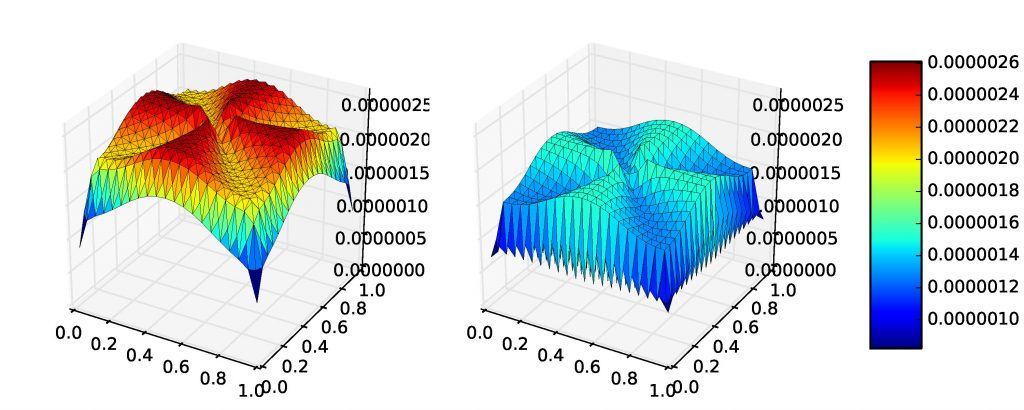Distribution of the global (left) and local (right) p-Laplace residual lifting, p = 1.5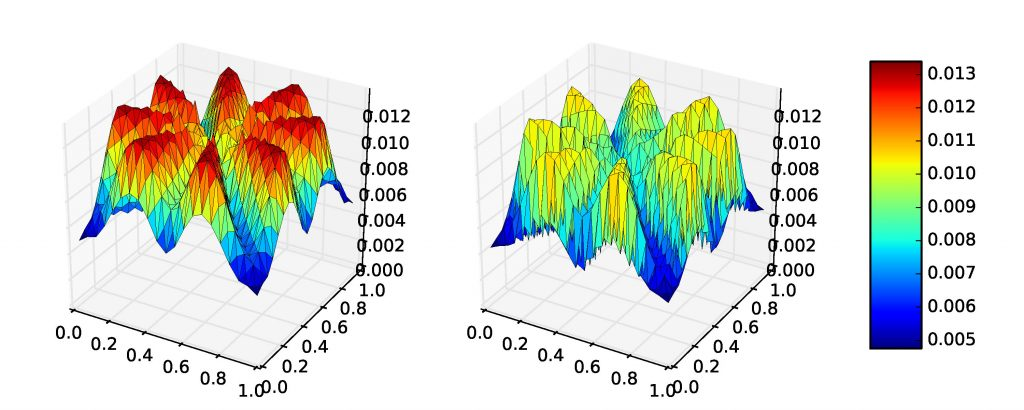Distribution of the global (left) and local (right) p-Laplace residual lifting, p = 10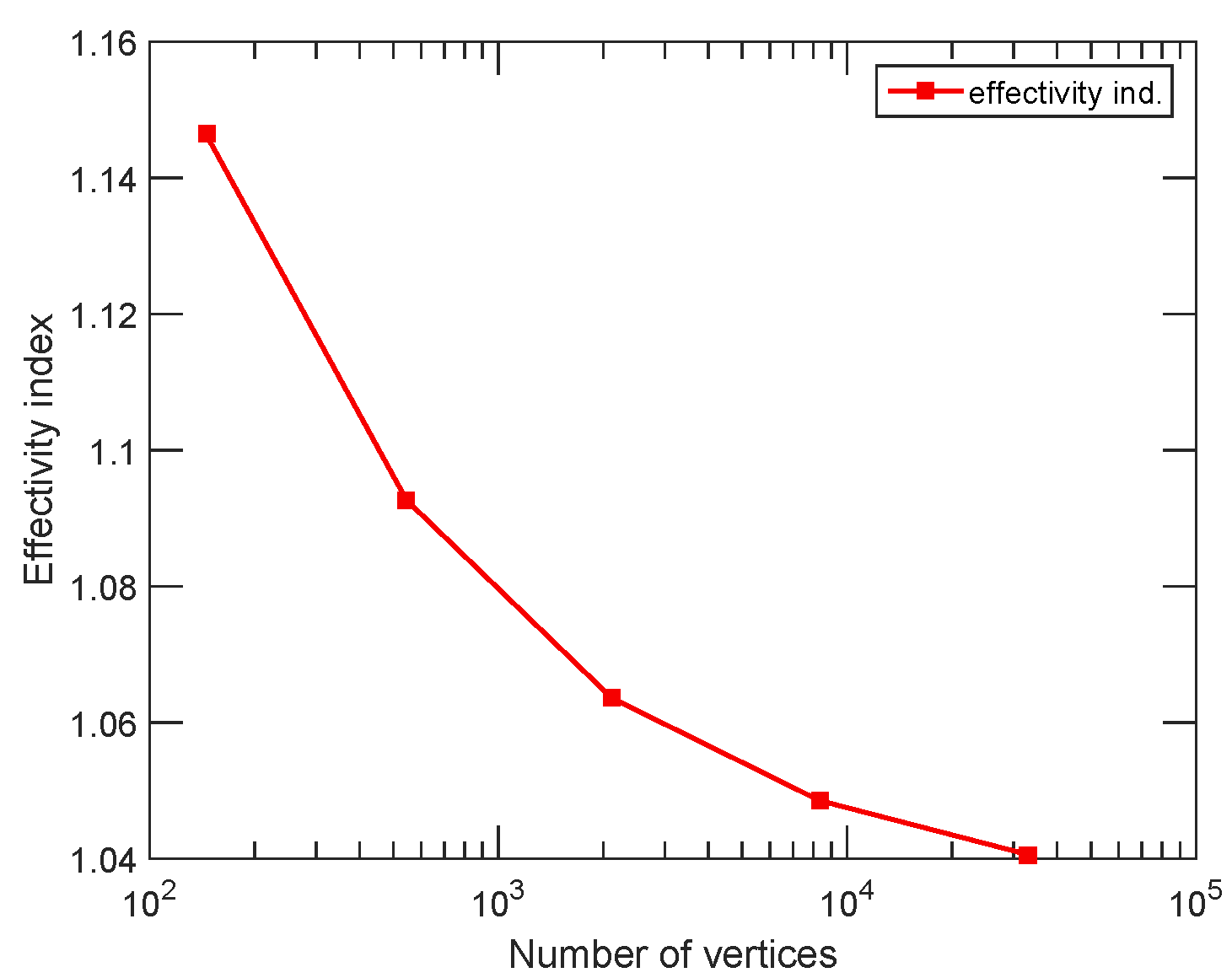Effectivity index, transmission problem, left subdomain diffusion -0.01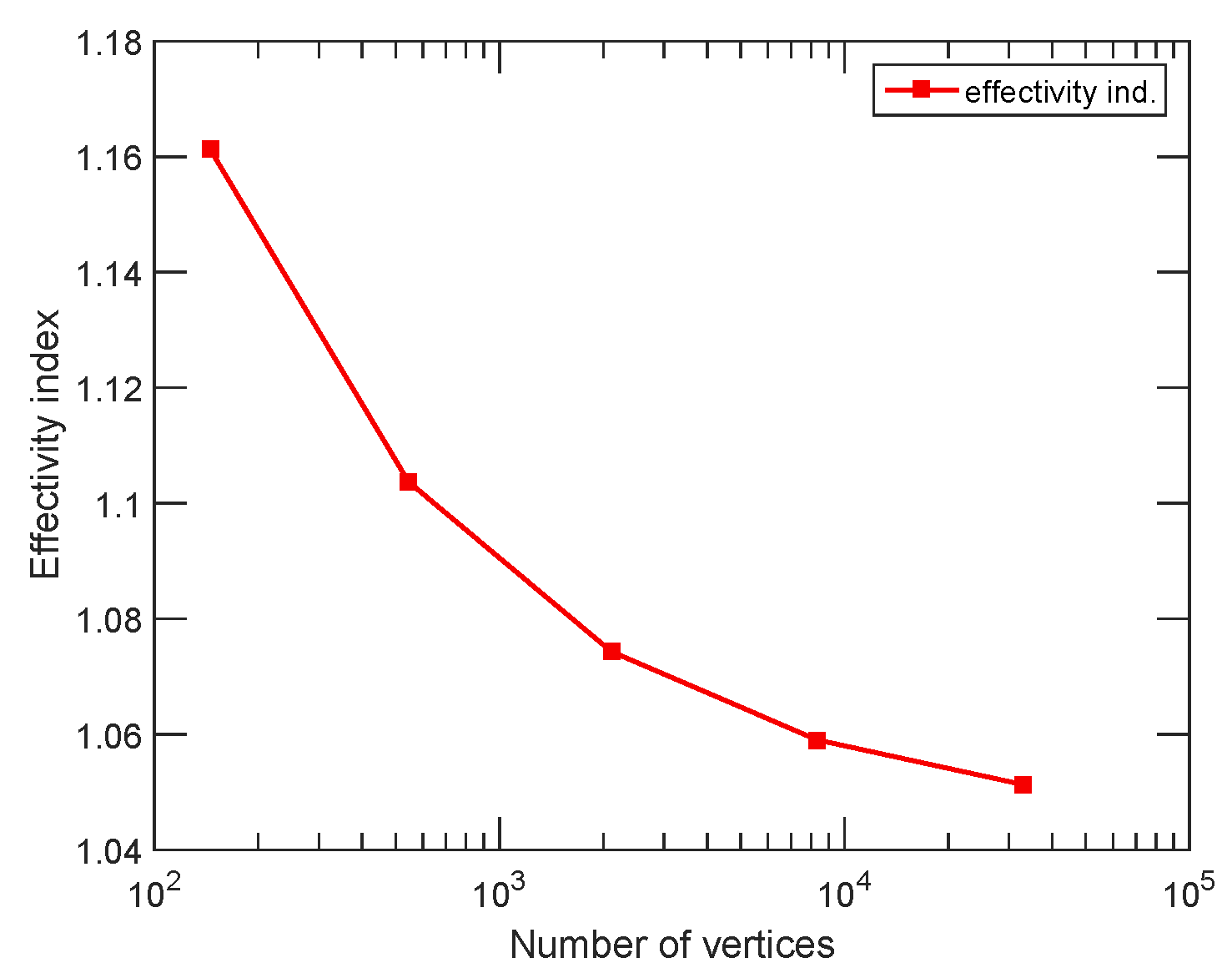Effectivity index, transmission problem, left left subdomain diffusion -0.33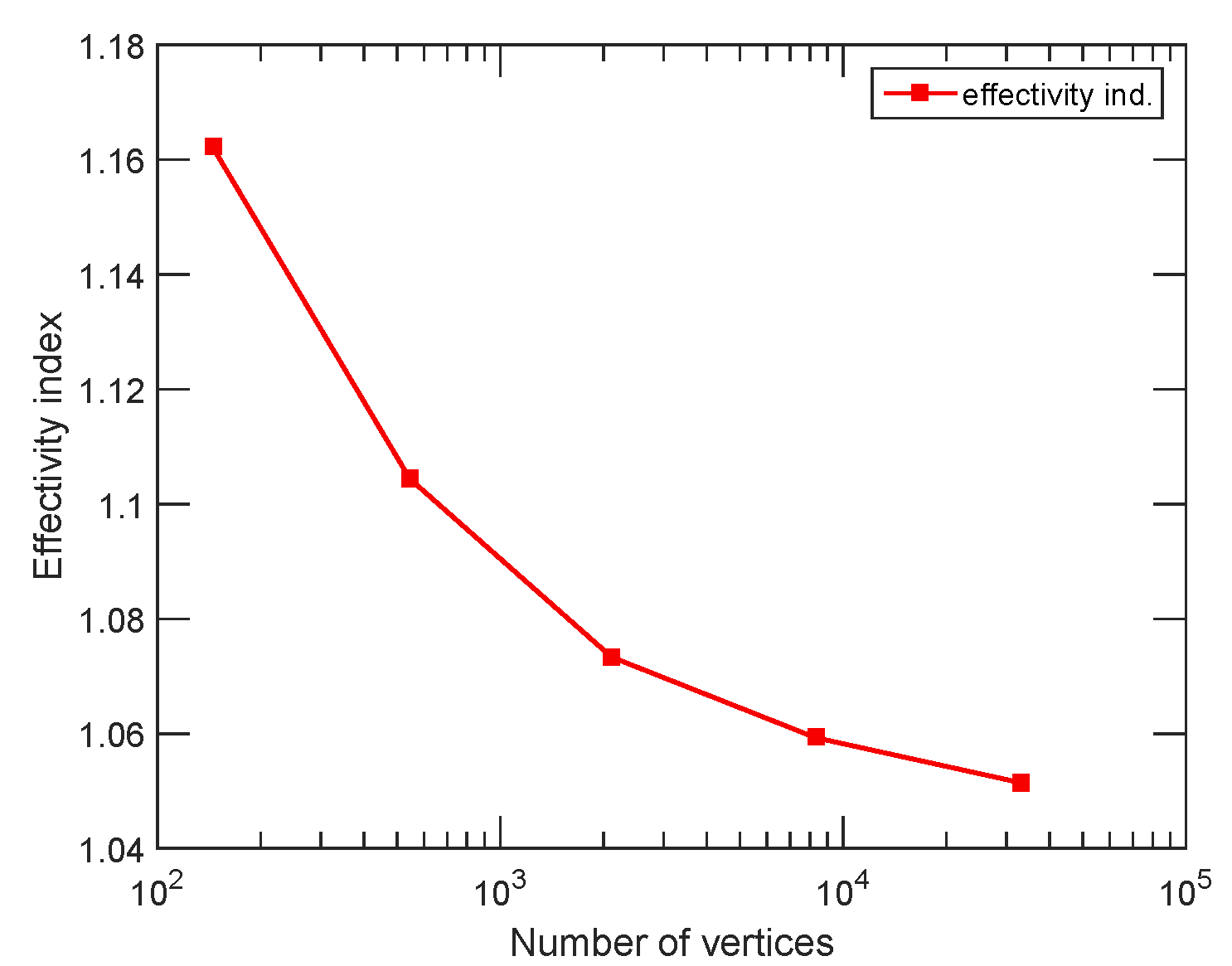Effectivity index, transmission problem, left left subdomain diffusion -0.99

Main results:

• dual norm of any bounded linear functional on the Sobolev space W01,p localizes under an orthogonality condition: equals the lq sum of local contributions;
• estimates taking into account the violation of the orthogonality condition;
• distance to the Sobolev space H01 localizes: equals the l2 sum of local contributions (no orthogonality condition);
• implies local efficiency and robustness of a posteriori estimates for nonlinear and non-coercive partial differential equations in divergence form;
• includes the case of inexact solvers.

Details:

H01 setting including nonconformity: paper (preprint) with Patrick Ciarlet, Jr., presentation

W01,p setting: paper (preprint) with Jan Blechta and Josef Málek, presentation